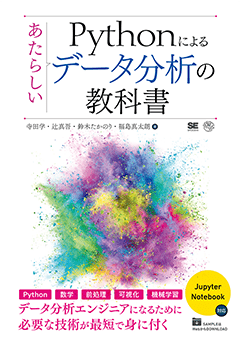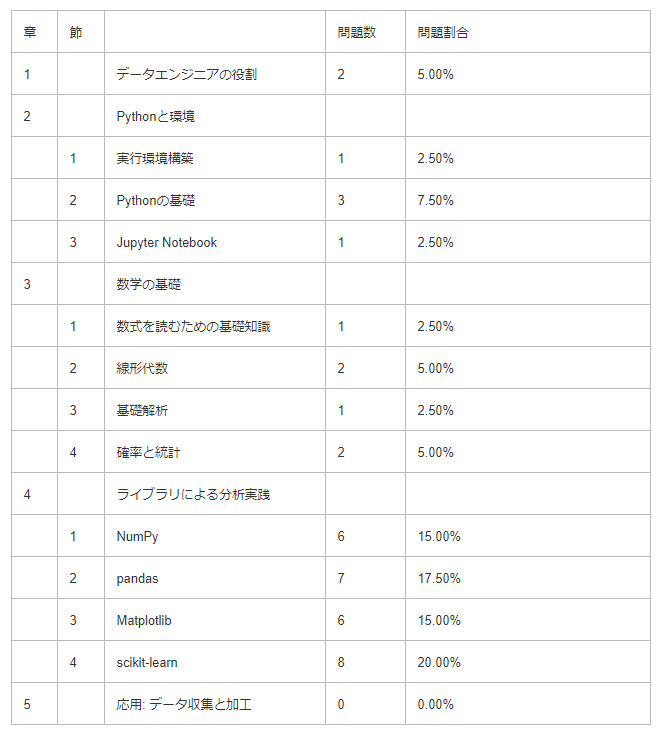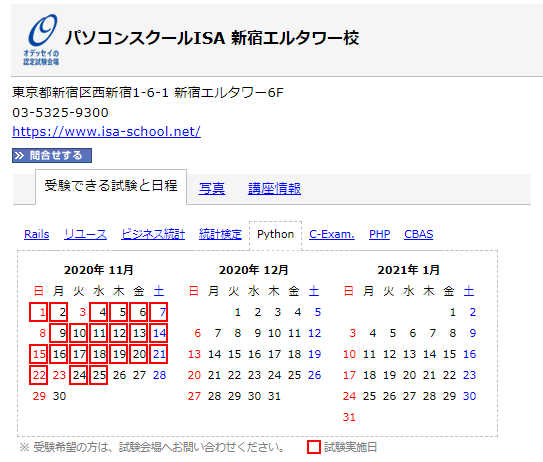# [For beginners] How to study Python3 data analysis exam

## ■ What is the Python3 data analysis test?

Name: Python 3 Engineer Certification Data Analysis Exam Summary: A test that asks the basics and methods of data analysis using Python Examination fee: 10,000 yen (excluding tax) Student discount 5,000 yen (excluding tax) Number of questions: 40 questions (all selection questions) Pass line: 70% correct answer rate Test Center: Odyssey Communications CBT Test Centers Nationwide Main teaching material: "New data analysis textbook using Python" (Shoeisha) Authors: Manabu Terata, Shingo Tsuji, Takanori Suzuki, Shintaro Fukushima (honorific title omitted)Held by the Python Engineer Development Promotion Association The exam is relatively for beginners.

For the exam, Python 3 Engineer Certification Basic Exam (Basic) There are two types: Python 3 Engineer Certification Data Analysis Exam (for practical use).

## ■ Get almost perfect score in 2 months from inexperienced

Started studying Python and machine learning from June of this year (2020) The other day (end of September), I took and passed the Python 3 Engineer Certification Data Analysis Exam.

The score was 975 out of 1000 (39 out of 40 correct answers).

I was inexperienced, but I was able to pass the exam with plenty of time in just two months. I would like to tell beginners how to study to make it easier to pass.

## ■ Study method

If I tell you only the conclusion first, once you have the necessary basic knowledge (Python, mathematics, machine learning) If you repeat the mock exam with the designated teaching material "New data analysis textbook using Python" I think I can pass with a margin.

By taking one lap (reading and copying sutras) to grasp the overall structure, and then repeating the mock exam and designated teaching materials This is because it is easy to understand the points at which the questions will be asked and to promote effective learning.

In my case, the designated teaching material was 3 laps of sutra copying, and I read 5 laps. The practice test was repeated 3 times, 3 times each.

## ■ Exam trends and countermeasures

Let's take a look at exam trends and countermeasures.

The range is as follows.[Problem format] ・ Basically, it is a question format of "choose one from four options". ・ Questions from the designated teaching material "New data analysis textbook using Python"

【difficulty】 ・ Python basics: Only basic knowledge, no problem if you actually copy and understand the code ・ Foundations of mathematics: Even if you can't solve the problem, there is no problem if you understand the definitions such as \$ \ pi \$ and Napier number \$ e \$. ・ Environment construction: There is no problem if you understand the contents of the textbook and memorize it. ・ Numpy ～ Scikit-learn: There is no problem if you understand by copying sutras.

## ■ Specific learning steps

Considering the specific learning steps, I think the following is good.

① Solidify the basics of Python ② Solidify the basics of mathematics (differential integration, linear algebra, probability statistics) ③ Acquire basic knowledge of machine learning ④ Read the designated teaching material book once and copy the sutras. ⑤ Take a mock test (1st or 2nd) ⑥ Repeat weak points with designated teaching materials ⑦ Take the mock exam (3rd) ⑧ Take a practice test (DIVE INTO EXAM)

• I think that there are individual differences in the degree of knowledge that each person has. Start learning from the steps that suit you.

## ■ Reference books / learning sites to use

★ is what I used.

① Solidify the basics of Python

◎ In many cases, learning sites are easier to study than books when studying coding.
② Solidify the basics of mathematics (differential integration, linear algebra, probability statistics)

Book for understanding mathematics for artificial intelligence programming (Author: Aidemy representative) ・ Easy learning Mathematics for understanding machine learning
③ Acquire basic knowledge of machine learning

Book for understanding mathematics for artificial intelligence programmingEasy learning Mathematics for understanding machine learningAidemy (Introduction to Machine Learning)
④ ⑥ Read the book of the designated teaching materials once and copy the sutras

◎ I highly recommend this book as it is a bridge from Python to machine learning. The content is neat and easy to read.
⑤ ⑦ Take a mock test (PRIME STUDY)

PRIME STUDY
⑧ Take a practice test (DIVE INTO EXAM)

## ■ Examination venue

Select the desired test site from Odyssey CBT. After that, move to the site of each venue and adjust the schedule to complete the reservation.

If you are in Tokyo, we frequently carry out tests as shown below. (As of 11/11/2020)## ■ Finally

In this article, I have told you about the study method based on my actual experience. I hope it helped you even a little.

Thank you for reading.